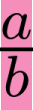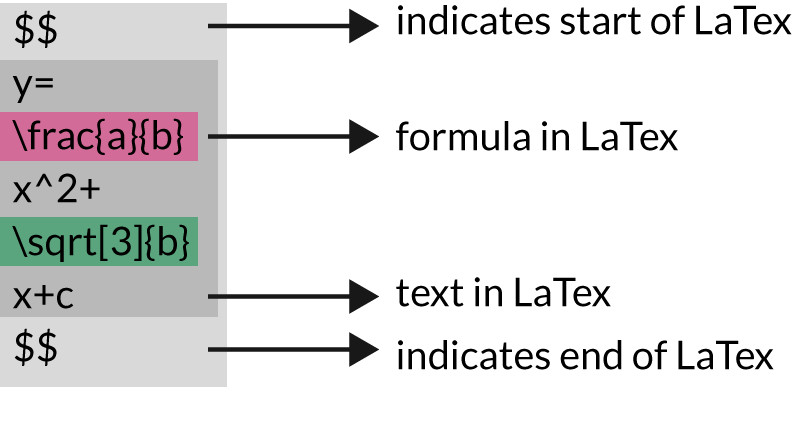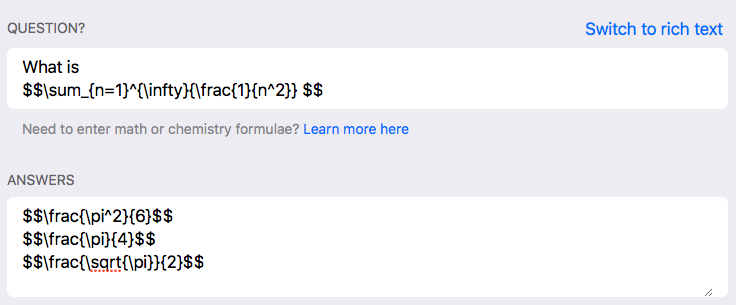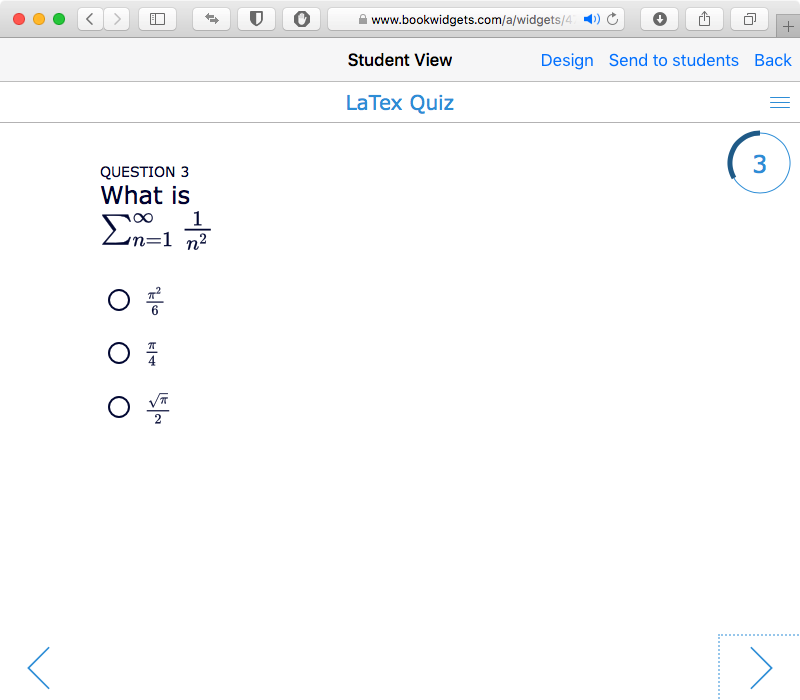# How to use LaTeX to spice up your math lessons - 3 Clever lesson ideas

Not a math or science teacher? Then I’m sure this could look a little bit (read: a lot) like Chinese. If you are a math teacher, you’ll love this! There are now a few apps that support LaTeX, which makes it easier for you to teach math with the visually correct formula’s.

But first…

## What is LaTeX?

Pro tip: pronounced «Lah-tech»

It all started in 1977. TeX was created by Donald E. Knuth, designed for typesetting mathematical formulas and texts. LaTeX, created by Leslie Lamport, is a document preparation system based on TeX. LaTeX is intended to provide a high-level language that accesses the power of TeX in an easier way for writers.

LaTeX is a markup language. You’re no longer using a WYSIWYG (What You See Is What You Get) interface to design formulas, but you now use simple text commands. This is the main disadvantage of LaTeX: you will not immediately see how the output looks, and you need to understand the syntax.

On the other hand, complex formulas can be typeset correctly in a mathematical way, and the syntax is easy enough to learn and understand. This is why LaTeX is widely used in the academic world for scientific documents.

## Educational apps supporting LaTeX

BookWidgets already supported LaTeX from its early years. We always found it to be the easiest way to accurately define and typeset mathematical and scientific formulas in our widgets or exercises.

Now that Apple released an update for iWorks to support LaTeX in Pages, Numbers and Keynote, it seemed like a good time to write a few words on how LaTeX works in BookWidgets.

Not only iWorks, but also iBooks Author already has support for LaTeX. And because our widgets can beautifully reside inside an iBook, it gives a killer combination for the crazy math and science teachers out there.

## LaTeX in BookWidgets: Examples

### Use LaTeX in Flashcards

In order to use LaTeX in tools like BookWidgets and iWorks, you need to learn LaTeX first. Check out these LaTeX flashcards to become a LaTeX pro, or simply use them as mnemonic.

### Use LaTeX in a Quiz

If you want your students to work out some calculations digitally, it’s important your calculations have the right format. Using LaTeX in the quiz widget also allows you to create automatically graded quizzes.

### Use LaTeX in a Pair Matching game

Math and gaming are a nice combination, if you can visually pull it off. And you can! Check out this pair matching game, and match the calculations with their solution. Are you up for this challenge?

## How to use LaTeX in BookWidgets

LateX is supported in the following widgets:

You’re probably wondering if LaTeX is easy to configure in BookWidgets. Don’t worry, it’s not hard at all. Start with remembering these 2 special characters: \$ and \. You mark the start and end of the LateX with 2 dollar signs $$. For example: $$ 5+2 $$ LaTeX comes with a set of mathematical symbols (or commands). You mark the start of such a LaTeX command with a backslash \, for example: \lambda  Everything succeeding a backslash \ is part of the LaTeX command. If the command needs some parameters, add them between curly braces {}, for example: $$ \frac{a}{b} $$ This LaTeX symbol stands for a fraction (\frac) with numerator a and denominator b. The result looks like this:You can add as many math formulas as you like, one behind the the other. For example, $$ y=\frac{a}{b} x^2+\sqrt{b}x+c $$ will result inHere is the formula broken down in parts:In BookWidgets, you just have type down a text (in LaTeX) in a text area, and mark your LaTeX with$$, and that’s it!The resulting widget looks like this:## LaTeX symbols cheat sheet

Although, LaTeX can look daunting at first, it gives you the power to accurately display mathematical formulas in BookWidgets. Learn the syntax, browse our examples and you will see that it’s not that hard at all.

If this is the first time you here about LaTeX, you’ll probably need a cheat sheet to find out about all the LaTeX symbols. Even if you know about LaTeX, it can be hard to memorize. So, here’s something to keep close:

Bart Buckinx